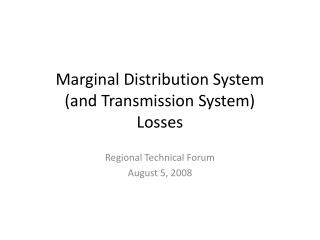# Marginal Distribution System (and Transmission System) Losses - PowerPoint PPT PresentationDownload PresentationMarginal Distribution System (and Transmission System) Losses

Marginal Distribution System (and Transmission System) LossesDownload Presentation## Marginal Distribution System (and Transmission System) Losses

- - - - - - - - - - - - - - - - - - - - - - - - - - - E N D - - - - - - - - - - - - - - - - - - - - - - - - - - -
##### Presentation Transcript

1. Marginal Distribution System (and Transmission System) Losses Regional Technical Forum August 5, 2008

2. Background • ProCost currently assumes: • At the April 29th RTF meeting, Jim Lazar proposed the use of a “marginal” distribution system loss ratio, which is higher than the normal distribution system loss ratio.

3. Review of the Original Proposal Percent Losses are proportional to the square of the load.

4. A Minor Adjustment Losses are proportional to the square of the load.

5. Are Marginal Losses are Higher than Total Losses? • Losses=I2R • Marginal Losses=dLosses=2IRdI (derivative) • Assuming line losses are caused entirely by I2R losses, voltage is held constant, and resistance is constant, “marginal losses as a percent of energy savings” is two times “total losses as a percent of total energy”. • See “proof” for more details:

6. However… • Is voltage really held constant? • If so, what does it take (\$ or energy or both) to hold it constant?

7. How To Incorporate into ProCost • Since marginal distribution system losses vary with both the system load shape and the measure savings shape, each measure’s load shape has a unique marginal distribution system loss ratio (marginal dist. system losses to energy savings).

8. ProCost (Continued) • However, we know line losses are not caused entirely by I2R losses. • This graph shows line losses at various mixes of I2R losses and “other losses” (assumption: marginal “other losses” do not vary with the load)

9. Decisions • Accept this analysis and program ProCost to generate measure-specific shaped “marginal distribution system loss ratios” to calculate busbar savings? • Components of Distribution System Losses (What Percent of the total losses are due to I2R losses?) • Applicability to Marginal Transmission System Losses • Seek further input?